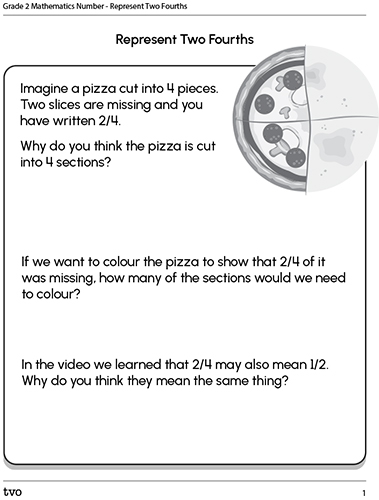# Minds On

## Activity – Missing pizza and egg sandwich

We are going to explore the video "Mystic Egg Pizza" to learn about fractions. In this episode of the Odd Squad, Olive and Otto help solve the case of missing pizza slices.

In the video we learned that two fourths of the pizza and two fourths of the egg sandwich were missing. How can we represent two fourths? Complete "Represent Two Fourths" in your notebook, or using the fillable and printable document below.

####Press the ‘Activity’ button to access Represent Two Fourths. Activity (Open PDF in a new window)

The image on the Activity document shows a pizza cut into 4 slices. Two (2) slices are missing. We can describe this as a fraction: we have two fourths of a pizza. Or: two fourths of the pizza are missing. Why do you think the pizza is cut into 4 sections? If we want to colour the pizza to show that two fourths of it was missing, how many of the sections would we need to colour? In the video we learned that two fourths may also mean one half.

Why do you think they mean the same thing?

# Action

## How can we describe a fraction?

When we want to describe a fraction, first we need to find out how many pieces there are in total. For example, the image of the pizza below has been cut into 8 slices. Suppose you have six friends that you will share this pizza with. How could you share this pizza equally with your six friends? How many slices would each friend get? Record your thinking using a method of your choice.There are 8 slices in the pizza - that is the whole.

Now we need to find out how many slices are left in our pizza. If there are 6 slices of the pizza taken out, how many slices are left?Each friend would get one slice of pizza with two slices left over. That is called the remainder - the amount left over after something has been shared equally.

## Let's share!

From the video, we learned that a pizza and a sandwich can be cut into equal parts. Let's practice sharing a pie equally with your friends. The pie is cut into 6 pieces. If you want to share the pie equally with 3 friends, how many pieces of the pie would each friend get? What if you want to share the pie with 3 friends? How many pieces of the pie would each friend get? What do you notice? Are there any pieces of the pie left over?# Consolidation

## How can we share a pizza equally?

I have 4 friends coming over and I ordered a pizza, which was not cut, so I ended up cutting the pizza. Did I do a good job at cutting the pizza for all of us? Why or why not?

Demonstrate how you would cut a pizza into 4 and how many different ways could you cut it equally. Draw a picture and write the fractions.

Here is a picture of how I cut the pizza.## Reflection

How do you feel about what you have learned in this activity? Which of the next four sentences best matches how you are feeling about your learning? Press the button that is beside this sentence.